# RD Sharma Class 10 Ex 15.1 Solutions Chapter 15 Areas Related To Circles

In this chapter, we provide RD Sharma Class 10 Ex 15.1 Solutions Chapter 15 Areas Related To Circles for English medium students, Which will very helpful for every student in their exams. Students can download the latest RD Sharma Class 10 Ex 15.1 Solutions Chapter 15 Areas Related To Circles pdf, Now you will get step by step solution to each question.

# Chapter 15: Areas Related To Circles Exercise – 15.1

### Question: 1

Find the circumference of a circle with a radius of 4.2 cm.

### Solution:

Given Data:

The formula to be used:

Circumference of a circle = 2

= 2 × 3.14 × 4.2 = 26.4 cm

Therefore, the circumference of the circle is 26.4 cm

### Question: 2

Find the circumference of a circle with area 301.84 cm2

### Solution:

Given Data:

Area = 301.84 cm2

We know that,

Area of a Circle = 301.84 cm2

= 3.14 × r = 9.8 cm

Circumference of a circle = 2

= 2 × 3.14 × 9.8 cm = 61.6 cm

Therefore, the circumference is 61.6 cm.

### Question: 3

Find the area of a circle whose circumference is 44 cm.

### Solution:

Given Data:

Circumference = 44 cm

We know that, Circumference of a circle = 2 cm

44 cm = 2 × 3.14 × r

r = 7 cm

Formula to be used:

Area of a Circle =

= 3.14 × 7 × 7

= 154 cm2

Area of a Circle = 154 cm2

### Question: 4

The circumference of a circle exceeds its diameter by 16.8 cm. Find the circumference of the circle.

### Solution:

Let the radius of the circle be = r cm

Therefore, Diameter (d) = 2r [radius is half the diameter]

We know that, Circumference of a circle (C) = 2

Given Data:

Circumference of a circle exceeds its diameter by 16.8 cm

C = d + 16.8

2 = 2r + 16.8      [d = 2r]

2 – 2r = 16.8

2r (- 1) = 16.8

2r (3.14 – 1) = 16.8

r = 3.92 cm

Circumference of a circle (C) = 2

C = 2 × 3.14 × 3.92

= 24.64 cm

Therefore, the circumference of the circle is 24.64 cm.

### Question: 5

A horse is tied to a pole with 28 m long string. Find the area where the horse can graze.

### Solution:

Given Data:

Length of the string 1 = 28 m

Area the horse can graze is the area of the circle in the figure shown with a radius equal to the length of the string.

We know that, Area of a Circle =

= 3.14 × 28 × 28 = 2464 m2

Therefore, the Area of the circle and the area the horse can graze is 2464 m2

### Question: 7

A steel wire when bent in the form of a square encloses an area of 121 cm2. If the same wire is bent in the form of a circle. Find the area of the circle.

### Solution:

Given Data: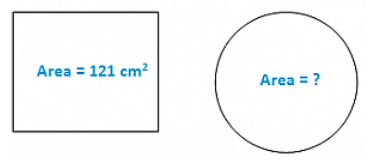Area of the square = 121 cm2

Area of the circle = πr2

We know that, area of a square = a2

121 cm2 = a2

a = 11 cm   [11= 121]

Therefore, each side of the square ‘a’ = 11 cm

We know that, the perimeter of a square = 4a

= 4 × 11 = 44 cm
Perimeter of the square = Circumference of the circle [in this case only]

We know that,

Circumference of a circle (C) = 2

4a = 2

2 = 44

r = 7 cm

We know that, Area of a Circle =

= 3.14 × 7 × 7 = 154 cm2

Therefore, the Area of the circle is 154 cm2

### Question: 7

The circumference of two circles is in a ratio of 2:3. Find the ratio of their areas.

### Solution:

Let the radius of two circles C1 and C2 be r1 and r2.

We know that, Circumference of a circle (C) = 2

Hence their circumference will be 21 and 22.

Also, their circumference will be in a ratio of = 21: 22

Given Data: circumference of two circles is in a ratio of 2: 3

Therefore, 21: 22 = 2: 3

Also, the ratios of their areas

= 4: 9

Therefore, ratio of their areas = 4: 9.

### Question: 8

The sum of the radii of two circles is 140 cm and the difference of their circumference is 88 cm. Find the diameters of the circles.

### Solution:

Let the radius of the circles be r1 and r2.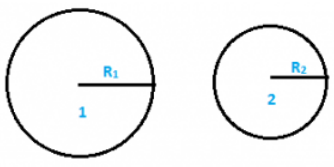Let the circumferences of the two circles be C1 and C2.

Given Data: We know that, Circumference of a circle (C) = 2

Sum of radii of two circles; r1 + r2 = 140 cm … (1)

Difference of their circumference,

C1 – C2 = 88 cm

21 – 2= 88 cm

2(r1 – r2) = 88 cm

(r1 – r2) = 14 cm

r1 = r2 + 14….. (2)

Substituting the value of rin equation (1)we have,

r+ r2 + 14 = 140

2r2 = 140 – 14

2r= 126

r2 = 63 cm

Substituting the value of rin equation (2)we have,

r= 63 + 14 = 77 cm

Diameter of circle 1 = 2r= 2 x 77 = 154 cm

Diameter of circle 2 = 2r= 2 × 63 = 126 cm

Therefore, Diameter 1 and diameter 2 are 154 cm and 126 cm

### Question: 9

A horse is placed for grazing inside a rectangular field 40 m by 36 m and is tethered to one corner by a rope 14 m long. Over how much area can it graze? (extra question)

### Solution: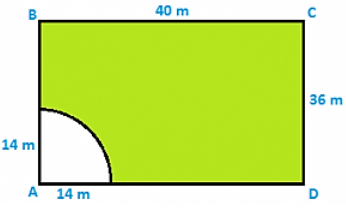The figure shows rectangular field ABCD at corner A, a horse is tied with rope length = 14 m. the area it can graze is represented A as shaded region = area of quadrant with (radius = length) of string

Area = 1/4 × (Area of circle)

= πr2 = 1/4 × 22/7 × 14 × 14

= (22 × 7) = 154 m2

Therefore, the area it can graze = 154 m2

### Question: 10

A sheet of paper is in the form of a rectangle ABCD in which AB = 40 cm and AD = 28 cm. A semicircular portion with BC as diameter is cut off. Find the area of the remaining paper.

### Solution:

Given Data: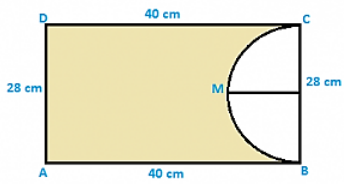Sheet of paper ABCD, AB = 40 cm and AD = 28 cm

CD = 40 cm and BC = 28 cm [since it is a rectangle]

Semicircle is represented as BMC with BC as the diameter.

We know that,

Area of a Circle = Area of the remaining (Shaded region) = (Area of rectangle) – (Area of semicircle)

= (AB × BC) – (0.5×) = (40 × 28) – (0.5 × 3.14 × 14 × 14)

= 1120 – 308

= 812 cm2

Therefore, the Area of the circle is 812 cm2

### Question: 11

The radii of two circles are 8 cm and 6 cm respectively. Find the radius of the circle having its area equal to the sum of the areas of two circles.

### Solution:

Given Data:

Radii of circles are 6 cm and 8 cm Area of circle with radius 8 cm = (8)2 = 64 cm2

Area of circle with radius 6cm = (6)2 = 36 cm2

Areas sum = 64 + 36 = 100 cm2

Let the radius of circle be x cm Area = 100 cm2

x2  = 100

x =√100 = 10 cm

Hence, x = 10 cm

### Question: 12

The radii of two circles are 19 cm and 9 cm respectively. Find the radius and area of the circle which has circumferences is equal to sum of the circumference of two circles.

### Solution:

Given Data:

Radius of the 1st circle = 19 cm

Radius of the 2nd circle = 9 cm

Formula used:

Circumference of 1st circle = 2(19) = 38 cm

Circumference of 2nd circle = 2(9) = 18 cm

Let radius of required circle be r cm Circumference of required circle = 2 = C1 + C2

2 = 38 cm + 18 cm

2 = 56 cm

Area of required circle = πr2 = 3.14 × 28 × 28 = 2464 cm2

Hence, the area of required circle = 2464 cm2

### Question: 13

The side of a square field is 10 cm. Find the area of the circumscribed and inscribed circles.

### Solution:

Circumscribed circle:

= 0.5 × 1.414 × 10 = 7.07 cm

Therefore, Radius of the circle, = 7.07 cm

We know that, Area of a Circle = 3.14 × 7.07 × 7.07 = 157.41 cm

Therefore, the Area of the Circumscribed circle is 157.41 cmInscribed circle: Radius = frac12∗side

= 1/2 × side

= 0.5 × 10 = 5 m

= 3.14 × 5 × 5 = 78.5 cm

Therefore, Area of the circle is 78.5 cm

### Question: 14

If a square is inscribed in a circle. Find the ratio of areas of the circle and the square.

### Solution:

Let side of square be x cm inscribed in a circle.Given Data:

Radius of circle (r) = 1/2 (diagonal of square)

= 1/2(√2)

= x/2

We know that, area of a square = x2

Hence, obtained.

### Question: 15

The area of circle, inscribed in equilateral triangle is 154 cm2. Find the perimeter of triangle.

### Solution:

Let the circle inscribed in the equilateral triangle be with a centre O and radius r.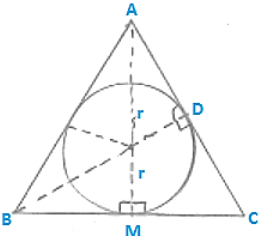Formula used:

We know that, Area of a Circle = but the given that area is 154 cm2.

3.14 × r2 = 154

= 7 × 7 = 49

r = 7 cm

From the figure shown above, we infer that at point M, BC side is tangent and also at point M BM is perpendicular to OM.

In equilateral triangle, the perpendicular from vertex divides the side into two halves.

BM =1/2

After solving the above equations we have,

Hence the perimeter to be found is 42√3 cm.

### Question: 16

A field is in the form of the circle. A fence is to be erected around the field. The cost of fencing would to Rs. 2640 at rate of Rs.12 per meter. Then the field is to be thoroughly plowed at cost of Rs. 0.50 per m2. What is the amount required to plow the field?

### Solution:

Given Data:

Total cost of fencing the circuit field = Rs. 2640

Cost per meter fencing = Rs 12

Total cost of fencing = circumference × cost per fencing

2640 = circumference × 12

Therefore, Circumference = 220 m

Let radius of field be ‘r’ m Circumference of a circle (C) = 220

2 × 3.14 × r = 220

r = 35 m

= 3.14 × 35 × 35 = 3850 m2

Cost of ploughing per m2 land = Rs. 0.5 × = 0.50 × 3850 m2 = Rs. 1925

Therefore, Cost of ploughing per m2 land = Rs. 1925

### Question: 17

A park is in the form of rectangle 120 m x 100 m. At the center of park, there is a circular lawn. The area of park excluding lawn is 8700 m2. Find the radius of the circular lawn.

### Solution:

Given Data: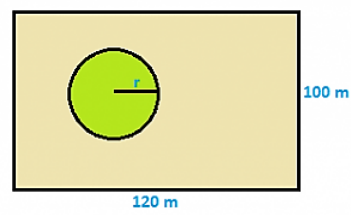Dimensions of rectangular park length = 120 m Breadth = 100 m

Area of park = I × b = 120 × 100 = 12000 m2

Let radius of circular lawn be r Formula used:

Area of circular lawn = Area of remaining park excluding lawn = (Area of park) x (Area of circular lawn)

8700 = 12000 – = 12000 – 8700

= 3300 m2

r = 32.4 m

Therefore, radius of circular lawn = 32.4 m

### Question: 18

A truck travels 1 km distance in which each wheel makes 450 complete revolutions. Find the radius of the wheel.

### Solution:

Given data:

Distance travelled = 1000 m Number of revolutions made = n = 450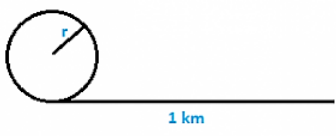Formula used:

We know that, Circumference of a circle (C) = 2

= 2 × 3.14 × r

Distance for 450 revolutions = (2 × 3.14 × r) 450

But we have been given with a distance of 1000 m, hence,

(2 × 3.14 × r) 450 = 1000

### Question: 19

The area enclosed between the concentric circles is 770 cm2. If the radius of the outer circle is 21 cm, then find the radius of the inner circle.

### Solution:

Given Data: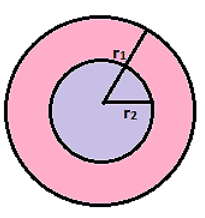Radius of outer circle = R1 = 21 cm

Radius of inner circle = R2

Area between concentric circles = Area of outer circle – Area of inner circle

Formula used:

We know that, Area of a Circle

= 770 cm= (R1– R22)

R1– R22   = 245

21– R22 = 245

R2 = 14 cm

Therefore, the radius of the inner circle is 14 cm.

All Chapter RD Sharma Solutions For Class10 Maths

I think you got complete solutions for this chapter. If You have any queries regarding this chapter, please comment on the below section our subject teacher will answer you. We tried our best to give complete solutions so you got good